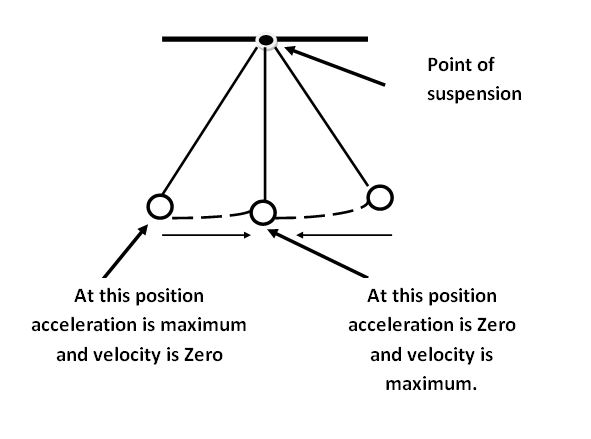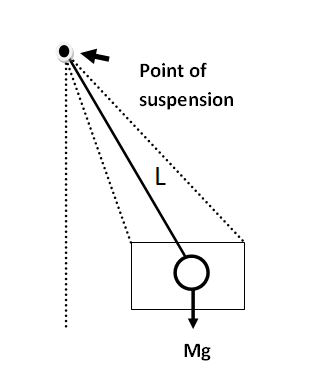# Difference Between Simple Pendulum and Compound Pendulum

• Last Updated : 15 Jun, 2022

Before going to know the difference between the simple pendulum and compound pendulum we have to know clearly about the simple pendulum and compound pendulum individually. A pendulum is a weight or body hanged from a fixed point so that it will swing back and forth. There are many different types of pendulums are there. Simple pendulum and Compound pendulum are the two types among the many different types of the pendulum.### What is a Simple Pendulum?

A simple Pendulum is used in clocks. Simple Pendulum simply has some weight called bob, on hanging the bob to the spring it will give a swing action because the gravity pulls downwards.

To find the period of a simple pendulum we have to assume that on a perfect plane pendulum will swing, Gravity remain constant, Friction between air and system remains constant, the pendulum arm does not bend or compress, and also it should be massless then only we can able to find the period of a simple pendulum.

The time period of a simple pendulum = Total time is taken/Number of Oscillations

or

T =2 π √(L/g)

where

• T is the time period,
• L is the length of the pendulum, and
• g is the gravity of the earth (g = 9.8m/s2).

Examples of a simple pendulum are: Cradle, Clocks, Bungee Jumping, etc.

Diagram of a simple pendulum is shown below.Simple Pendulum

• It is simple to use and Low installation cost.
• Safety operations are carried out by using this simple pendulum.

• A simple pendulum bob is not weighed less than much heavy point bob.
• The motion of the bob is not linear.
• Resistance and buoyancy are negligible.

### Applications of Simple Pendulum

1. On the Diving board, a simple pendulum is used.
2. In Earth quake-proof building simple pendulum is used.
3. In Bungee Jumping simple pendulum is used.
4. In musical instruments, a simple pendulum is used.

### What is Compound Pendulum?

A compound pendulum weights bar which is in swing mode and it freely oscillates about the horizontal access. The time period of the compound pendulum is measured from the center of oscillation to the pivot level. The compound pendulum is also known as the Physical pendulum.

Radius of oscillation of compound pendulum = I/(m × R)

where

• I is inertia,
• m is mass, and
• R is the distance between the center of mass and pivot value.

Time period of compound pendulum= 2 × π(√(I/mgR))

where

• g is the acceleration due to gravity.

Examples of compound pendulums are: weight distribution machine, sailing boats, farm tractors, etc.

General diagram of the compound pendulum is shown below.Compound pendulum

• Time can be calculated accurately by using a compound pendulum.
• The compound pendulum has more energy.

• The compound pendulum varies its behavior while moving around the earth.
• If the temperature changes time period of the swing also varies.

### Applications of Compound Pendulum

1. In farm tractors, a compound pendulum is used.
2. In sailing boats, compound pendulum is used.
3. In hockey sticks, a compound pendulum is used.
4. In golf, a compound pendulum is used.

### Sample Questions

Question 1: If the time period of a simple pendulum is 2.5 s, then determine its length.

We know that formula for a time period of a simple pendulum,

T=2 π √(L/g)

where,

L= length of the simple pendulum , g=gravity=9.8 m/s2 , given T = 2.5 s.

Substitute T, g in above formula we will get length of the simple pendulum

2.5 = 2 x 3.14 √L/9.8

2.5 / (2 x 3.14) = √L/9.8

0.3980 = √L/9.8

Squaring on both sides,

0.158 = L/9.8

L = 1.5484 m

Question 2: What is the frequency of a simple pendulum that has a length of 2 m and gravity is 10 m/s2

Given that length of the pendulum L = 2 m

and Gravity (g)=10m/s2

We know that the time period formula of a simple pendulum is,

T=2 π √(L/g)

Therefore,

Frequency (f)=1/T

Substitute L, g in above equation we get period(T)

T = 2 x 3.14 (√(2/10))

= 6.28 x (√(0.2))

= 6.28 x 0.447

= 2.80 s

But, Frequency is inversely proportional to time period so

f = 1/T

= 1/2.80

= 0.3571 Hz

Question 3: Gravity on the moon is 1.6 m/s2. Find the length of the simple pendulum if the time period is given 3 s.

Given that gravity on moon (g)=1.6 m/s

Time period (T)= 3 seconds

To find length of the pendulum we know that

T = 2 π √(L/g)

3 = 2 x 3.14 √L/1.6

(3/6.28)2=L/1.6

0.2275=L/1.6

L = 0.364 m

Question 4: The frequency of the simple pendulum is 0.8 Hz. What is the gravity of a simple pendulum if the length of the pendulum is 2 m?

Given that frequency of the simple pendulum (f)=0.8 Hz.

Length of the simple pendulum (L) = 2 m

To find gravity of the simple pendulum we know that,

T = 2 π √(L/g)

We know that T=1/f

1/f=2 π √(L/g)

L/g=(2 π f)2

g=L/(2 π f)2

= 2/(2×3.14×0.8)

=2/5.024

=0.39 m/s2

Question 5: Find the time period of a compound pendulum having a mass moment of inertia = 150 Kg-m2, the mass of 15 Kg, and the gravity lies at a point of 22 cm.

Given that, I = 150 Kg-m2

Mass (m) = 15 kg

R = 22 cm = 0.22 m

We know that time period of compound pendulum,

T=2xπ(√(I/mgR))

= 2×3.14(√(150/(15×9.8×0.22))

=6.28√(150/32.34)

=6.28√(4.6)

=13.46 s

My Personal Notes arrow_drop_up